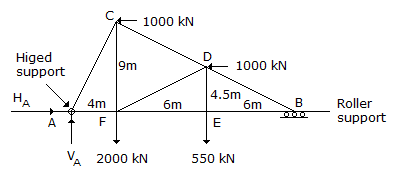# Civil Engineering - UPSC Civil Service Exam Questions

46.
A truss ABC, carries two horizontal and two vertical loads, as shown in the figure. The horizontal and vertical components of the reaction at A will beHA = 1000 kN ; VA = 1706.25 kN
HA = 2000 kN ; VA = 2550 kN
HA = 1000 kN ; VA = 2550 kN
HA = 2000 kN ; VA = 1706.25 kN
Explanation:
No answer description is available. Let's discuss.

47.
Contour interval on a map sheet denotes
vertical distance of contour lines above the datum plane
vertical distance between two successive contour lines
slope distance between two successive contour lines
horizontal distance between two successive contour lines
Explanation:
No answer description is available. Let's discuss.

48.
Which one of the following is the correct statement ? A particle in simple harmonic motion while passing through the mean position will have
minimum kinetic energy and minimum potential energy
maximum kinetic energy and maximum potential energy
maximum potential energy and minimum kinetic energy
maximum kinetic energy and minimum potential energy
Explanation:
No answer description is available. Let's discuss.

49.
What are the phenomena of global warming and acid rain formation attributed to ?
SO2 and CO2 respectively
CO and SO2, respectively
CO2 and SO2, respectively
CO and CO2, respectively
Explanation:
No answer description is available. Let's discuss.

50.
The following observation relate to designing of laced column :
1. Single lacing systems on opposite planes shall preferably be in the same direction so that one is the shadow of the other.
2. Lacing bar should only be a flat.
3. The slenderness ratio of the lacing bars for compression shall not exceed 180.
4. Laced compression members are to be provided with tie plates of ends.
Of these observations :
1, 2, 3 and 4 are correct
1, 3 and 4 are correct
2 and 3 are correct
1 and 4 are correct